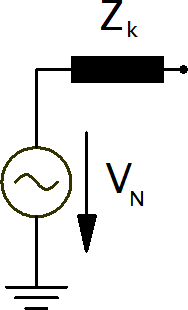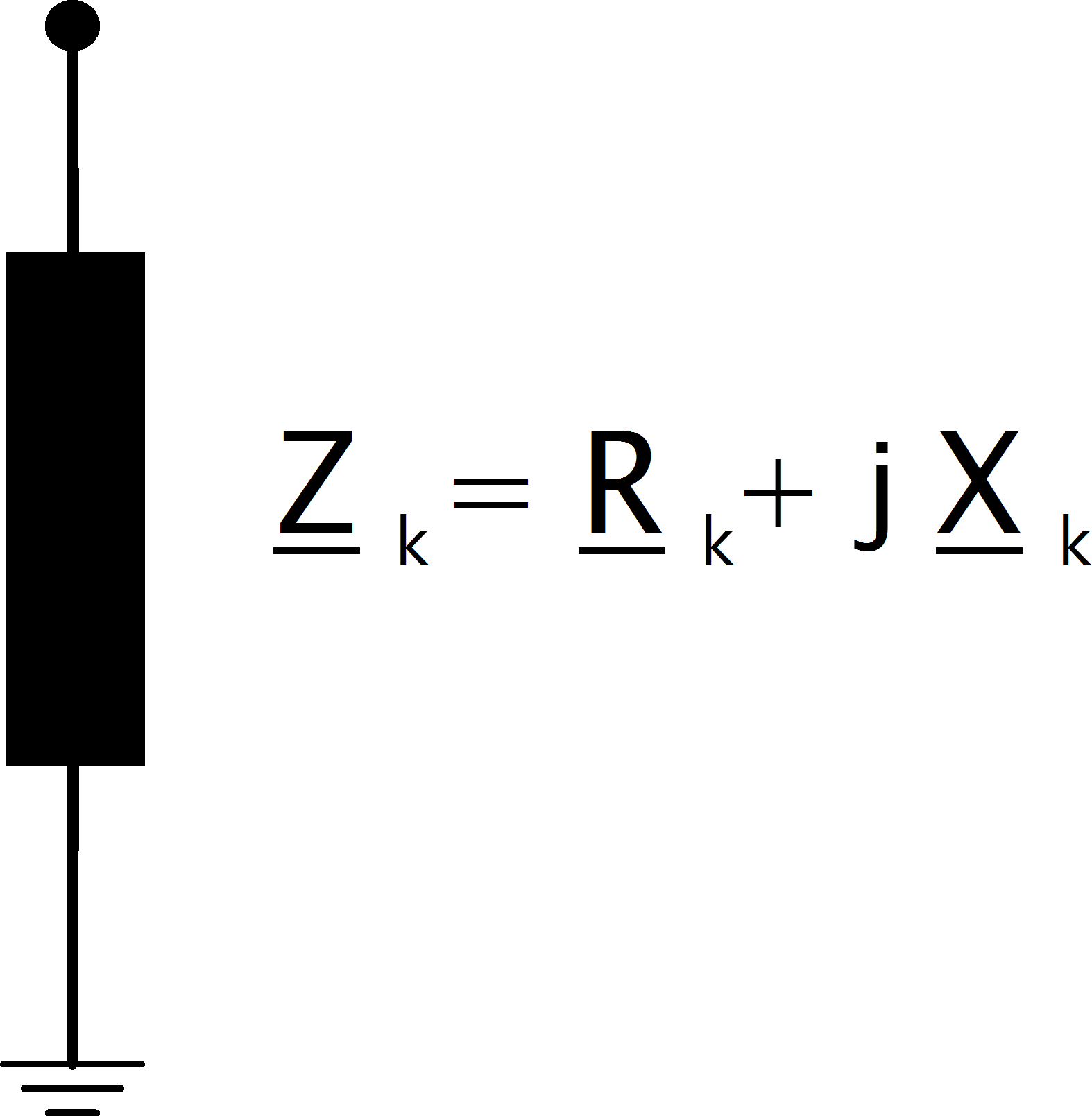# Voltage Source Elements

Voltage source elements are represented by their internal voltage source with an internal resistance $$Z_k$$:since the voltage source is moved to the fault location for with methodology of the equivalent voltage source, the bus elements can be reduced to a single shunt impedance:The contribution of loads and shunts are negligible according to the standard and therefore neglected in the short-circuit calculation.

## External Grid

When calculating maximum short-circuit currents, the impedance of an external grid connection is given as:

$\begin{split}z_{k, eg} =& \frac{c_{max}}{s\_sc\_max\_mva} \\[1em] x_{k, eg} =& \frac{z_{sg}}{\sqrt{1 + rx\_max^2}} \\[1em] r_{k, eg} =& rx\_max \cdot x_{sg}\end{split}$

where $$rx\_max$$ and $$s\_sc\_max\_mva$$ are parameters in the ext_grid table and $$c_{max}$$ is the voltage correction factor of the external grid bus.

In case of minimal short-circuit currents, the impedance is calculated accordingly:

$\begin{split}z_{k, eg} =& \frac{c_{min}}{s\_sc\_min\_mva} \\[1em] x_{k, eg} =& \frac{z_{sg}}{\sqrt{1 + rx\_min^2}} \\[1em] r_{k, eg} =& rx\_min \cdot x_{sg}\end{split}$

## Asynchronous Motor

Asynchronous motors can be considered by setting the type variable of an sgen element to “motor”. The internal impedance is then calculated as:

$\begin{split}Z_{k, m} = \frac{1}{k} \cdot \frac{vn\_kv^2 \cdot 1000}{sn\_kva} \\ X_{k, m} = \frac{Z_{sg}}{\sqrt{1 + rx^2}} \\ R_{k, m} = rx \cdot X_{sg}\end{split}$

where $$sn\_kva$$ is the rated power of the motor, $$k$$ is the ratio of nominal to short circuit current and $$rx$$ is the R/X ratio of the motor. $$vn\_kv$$ is the rated voltage of the bus the motor is connected to.

## Synchronous Generator

Synchronous generators are considered with the short-circuit impedance of:

$\underline{Z}_{k, gen} = K_G \cdot (R''_d + jX''_d)$

The short-circuit impedance is calculated as:

$z_k = xdss$

The generator correction factor $$K_G$$ is given as:

$K_G = \frac{V_{N, gen}}{V_{N, bus}} \cdot \frac{c_{max}}{1 + x_{dss} \cdot sin(\varphi)}$

where $$V_{N, bus}$$ is the rated voltage of the bus the generator is connected to and $$V_{N, gen}$$ is the rated voltage of the generator which is defined by the parameter $$\text{sn\_kva}$$ in the gen table. The rated phasor angle $$\varphi$$ is given as:

$\varphi = arcos(cos\_phi)$

where $$cos\_phi$$ is defined in the gen table.